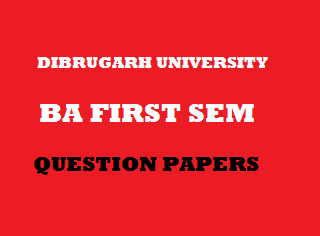## Friday, January 04, 20192013
(November)
ECONOMICS
(General)
Course: 101
(Microeconomic Theory)
Full Marks: 80
Pass Marks: 32
Time: 3 hours
The figures in the margin indicate full marks for the question

1. Answer the following questions/Fill in the blanks: 1x8=8
1. What do you mean by scarcity in economics?
2. In case of independent goods, the cross elasticity of demand will be ____.
( negative / positive / zero )
1. What is selling cost?
2. Indifference curve analysis is based on ____ marginal rate of substitution.
( diminishing / increasing / constant )
1. Give the meaning of normal profit.
2. What is ‘reserve price’?
3. Which type of wages determines the standard of living of workers?
4. What do you mean by insurable risks?
2. Write short notes on any four (within 150 words each): 4x4=16
1. Micro dynamics.
2. Cardinal utility and Ordinal utility.
3. Relationship between Average Revenue and Marginal Revenue.
4. Characteristics of monopolistic competitive market.
5. Collective bargaining.
Answer the following questions (within 500 words each):
3. (a) Define microeconomics. Explain the concept of microstatics with the help of diagram. 2+9=11
Or
(b) Explain the usefulness of studying microeconomics. 11
4. (a) Explain consumer’s equilibrium with the help of indifference curves. 11
Or
(b) What do you mean by income elasticity of demand? Explain with diagram the different types of income elasticity of demand. 2+9=11
5. (a) Define money cost, real cost and opportunity cost. Explain with diagrams the shape of AFC and AVC curves. 6+5=11
Or
(b) Distinguish between: 5+6=11
1. Fixed cost and Variable cost.
2. Short-run Marginal Cost and Short-run Average Cost.
6. (a) What is long-run price? Explain with diagrams the long-run price determination process of industry and firm under perfect competition. 2+10=12
Or
(b) (i) Define total revenue, average revenue and marginal revenue. 6
(ii) Explain the equilibrium of a firm with the help of marginal revenue and marginal cost. 6
7. (a) what is quasi-rent? Explain the concept of quasi-rent with diagram. 2+9=11
Or
(b) Explain Keynesian theory of interest. 11

***